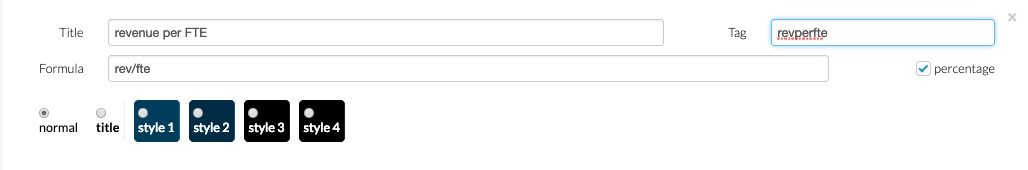# Formulae in reports

You can use a range of formulae in your reports, and these can be built in different ways!

When you are in the report building page, simply drag the '+ NEW FORMULA' box to the area that you want to compute the formula.You will then see a pop-up box where you can build your formula.

###### Referencing accounts

This is where you directly reference the account numbers you want in your formula- this will be specific to your chart of accounts.

For example: Days payabale

((#240000__240019)*-1)/(#5)*365

• #5 means that all the amounts for all the accounts beginning with 5 have been added together
• #240000__240019 means that all the accounts 240000 through to 240019 have been added together (note that the symbol here is two underscores, _ and _, with no space between them)

#### Referencing tags

You can also use ‘tags’ for groups of accounts. These tags can then also be used in calculations. Do watch out, however, for typos and capital letters: tags are case-sensitive.

Just a reminder on how to make tags: Simply select the pencil next to any line in your report and you can create a tag.

#### Possible operations:

The function signs are the same as in other applications:

- subtract

/ divide

* multiply

# this will sum all numbers

__ (2 underscores) this will compute the range

Be careful! If you want to make a calculation involving figures, make sure the separator for decimals is a decimal point, not a comma (so use 1200.23 rather than 1.200,23 or 1200,23).